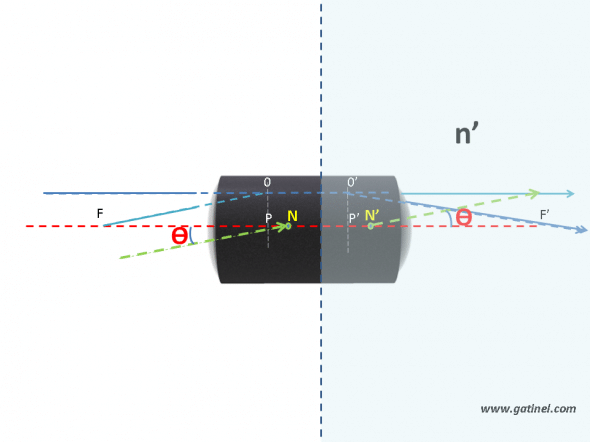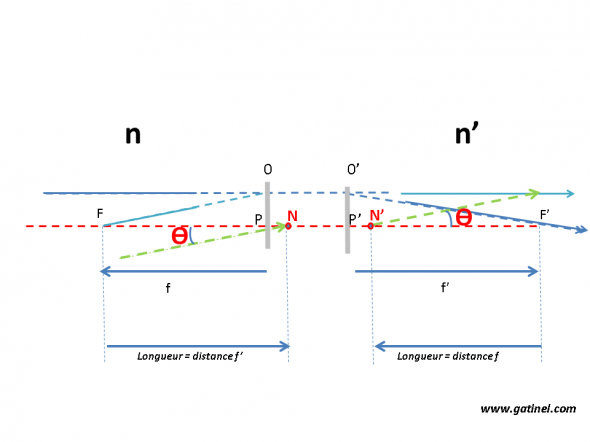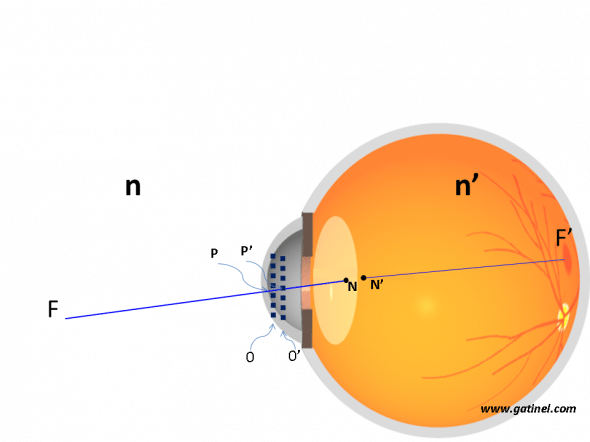+ +

# Point node (nodes)

The nodal points of an optical system are rated cardinal points N and not are two combined points (one - point node image is the image of the other, the point node object) they are located on the optical axis, and such as to any incident Ray passing through the point node object, match an emerging Ray through the nodal point image and parallel to the incident Ray. When the optical system is located in an environment where the index of refraction of the Middle image and the environment object are identical, the nodal points are confused with the main points.

The anatomic proximity of two nodes allow their merger in a 'single nodal point' for calculations at theVisual acuity (angular resolving power). The nodal point is located near the posterior crystalloid, and we generally choose a distance of 16.7 mm between the nodal point and the fovea for calculations.When the middle where emerges the RADIUS has a different price reduction index from the middle of the incident Ray, we can set another pair of (distinct from the main points) points, called nodes, and for which the angular magnification is 1: the incident rays directed to the nodal point of entry give each emerging RADIUS which forms the same angle with the optical axis of the incident Ray. The distance NN' is equal to the distance PP 'and one can show that the FP distance is equal to the distance didn't F'.

Geometric relations between the nodal points and the other cardinal points are represented in the following diagram:In algebraic value (positive distance to the right and negative to the left) we can ask: FN = P 'F' = f' F 'Not = F 'P' + P' doesn't = NF + fn = PF = f fn = FP + FN = F' no + P 'F' = P 'Doesn't = f + f'

In photography, the rear nodal point is one around which rotate the device if you want to take several shots without that live their position with respect to the plan focal image moves.

In the case of the human eye, the Middle image is the vitreous (n = 1336), which is different from the middle of the air (n = 1). For a standard model look, the nodal points its located on both sides of the posterior capsule of the lens, "backward" (in the sense of the propagation of light) key points P and P'For a look at rest (no accommodation), the nodal points N and are located on both of the posterior crystalloid (posterior capsule of the lens). The distance didn't F ' is estimated at 16.7 mm. The P 'F' distance is estimated close to 22.3 mm, and the distance of PN = P' doesn't = 5.6 mm. F is the home object of the eye: its sharp image would be formed to infinity (if the retina is not was on the way to the light Ray).